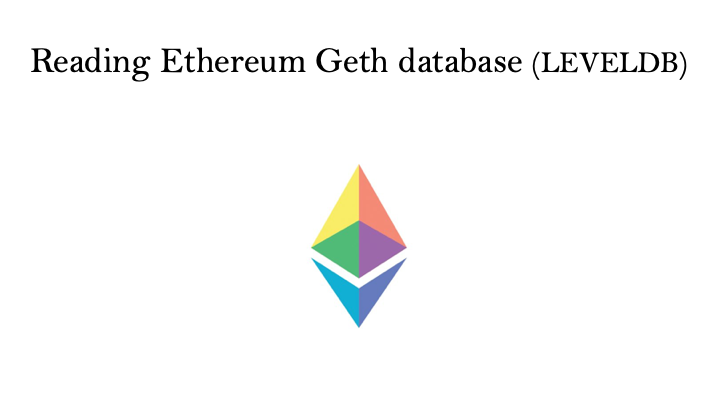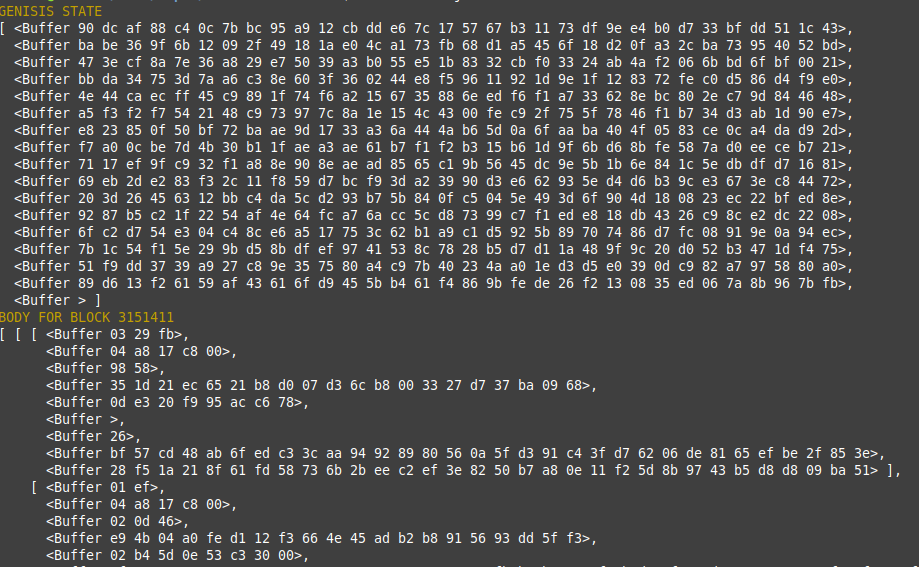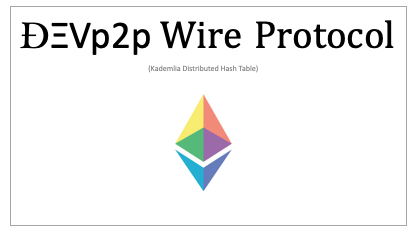### Reading Ethereum Geth database (LEVELDB)

Geth stores all the blockchain data in LevelDB. LevelDb is a simple database which stores value in key-value pair. Geth stores all the levelDB related data files in this directory "\$DATADIRECTORY/geth/chaindata", where "DATADIRECTORY" is path to Ethereum data directory. We normally do not need to access these data files because geth provides many utilities to interact with underlying data structure. But, just to understand how geth stores data, let's hack a little bit.

Let's have a look at this file from geth GitHub repo

``````headerPrefix=k []byte("h") // headerPrefix + num (uint64 big endian) + hash -> header

``````

As you can see in the file, key to store header is buffer concatenation of ("h"+blocknumber+blockhash) like below

``````const headerKey = (n, hash) => Buffer.concat([Buffer.from('h'), bufBE8(n), hash])
``````

Similarly for receipt and bodykey

``````const bodyKey = (n, hash) => Buffer.concat([Buffer.from('b'), bufBE8(n), hash])
const headerKey = (n, hash) => Buffer.concat([Buffer.from('h'), bufBE8(n), hash])
const recptKey = (n, hash) => Buffer.concat([Buffer.from('r'), bufBE8(n), hash])

``````

Whereas state tries key does not have prefix , it can be retrieved taking state root as key.

I have put together some sample code for Demo using some of ethereumJS library. Here is the list of libraries I have used.

``````const level = require('level')
const rlp = require('rlp')
const ethUtil = require('ethereumjs-util')
const BN = ethUtil.BN

``````

"level" is a library to interact with LevelDB database.
"rlp" , Geth install all the data encoded as rlp , so I have used to decode the data after retrieved from levelDb
"ethereumjs-util" is ethereumjs utility library..

``````
const bufBE8 = n => n.toArrayLike(Buffer, 'be', 8)
const bodyKey = (n, hash) => Buffer.concat([Buffer.from('b'), bufBE8(n), hash])
const headerKey = (n, hash) => Buffer.concat([Buffer.from('h'), bufBE8(n), hash])
const recptKey = (n, hash) => Buffer.concat([Buffer.from('r'), bufBE8(n), hash])

``````

Above is the key format which geth uses to store data and below is the sample code to retrieve body of block using "blocknumber" and "blockhash".

``````ReadLevelDB.prototype.getBody=function(blockNumber,blockHash,cb){

this.db.get(bodyKey(new BN(blockNumber),ethUtil.toBuffer(blockHash)),{
keyEncoding: 'binary',
valueEncoding: 'binary'
}, function (err, value) {
cb(err,rlp.decode(value))

})
}

``````

I have committed codes on github with instruction on how to run it for your reference.

Here is the output when you run index.js file from repo.GITHUB REPO

If you find this article helpful, you may show your appreciation by sharing it. Also, you may reach me at hello.bitwarrior@gmail.com with your comments, questions or suggestions of new topics that you would want to be covered at EtherWorld.co.

Read more articles at EtherWorld's collection of Good Read on Blockchain & Cryptocurrency.____________________________________________________________________________________________________